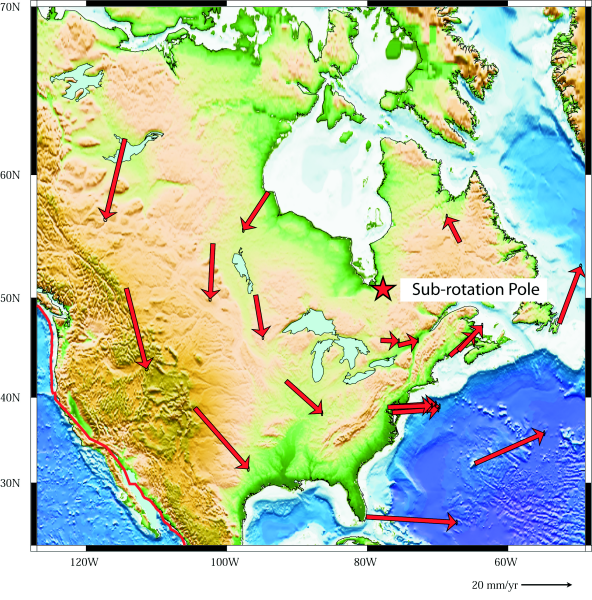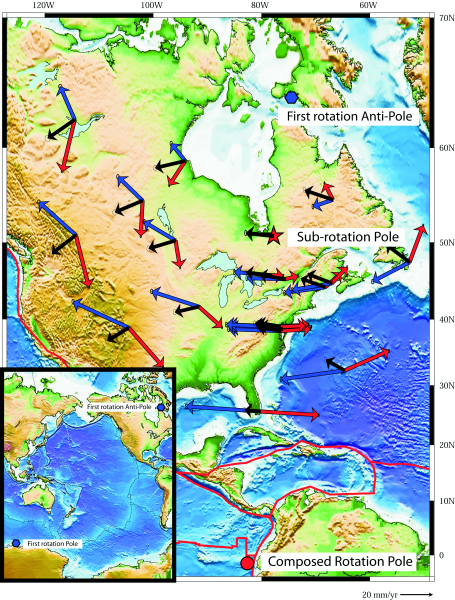## The sub-rotation of the North America and discussion

The application of the equations (5) and (6) to the North American plate is 1stly a test for the analytical exactness of the two-rotation plate motion model. There is no clear geodynamical evidence (as is the case for the example of Iberia) that suggests the North American plate motion is composed by two contemporaneous rotations, though Doglioni (1990) suggests such a sub-rotation of the North American plate can be justified.

This plate was chosen also because it has the largest number of GPS stations in the global network and their velocities are reported in an absolute reference frame, ITRF2000 (Altamimi et al. 2002), and this is useful for comparison with model results. In this reference frame a no-net-rotation condition (NNR) is conventionally imposed.

Using the angular velocities of the present-day plate kinematic and deformation model (APKIM2002) (Drewes and Meisel 2003), we suppose a priori that the North American 1st rotation pole is the APKIM2002 Pacific rotation pole located at (-64.30 ± 0.18) °N and (105.52 ± 1.15) °E with an angular velocity `ωr` = (0.6588 ± 0.0039) °.Myr-1.

To align the two-rotation plate motion to the GPS velocities distribution (Heuin et al. 2004) it is necessary to introduce a contemporaneous sub-rotation on the 1st rotation and the result is a sub-rotation pole located at (50.78 ± 0.06) °N, which is not far from the rotation pole of Stein and Sella (2002) and (-77.78 ± 0.50) °E with a sub-rotation angular velocity `ωs` = (0.7594 ± 0.0069) °. MYr-1 . With these two sets of motion parameters, i.e., 1st rotation and sub-rotation, we applied equation (3) then (4) for 20 selected intraplate space geodesy stations, far from the diffusely deformed western North America plate margin. The results are shown in Table 1.

Figure 4 and Figure 5 show respectively and separately the 1st rotation of the GPS stations about the 1st rotation pole and their sub-rotation about the sub-rotation pole. When we consider only the sub-rotation, the sub-rotation pole is fixed (Figure 5), but if there is also the 1st rotation, the sub-rotation pole moves along a parallel of the 1st rotation pole (Figure 4).

Figure 4. Sub-rotation of the North AmericaAssuming that the first rotation of the North American plate is along the pacific trend (see text), the selected GPS stations of the North American plate rotate firstly about the APKIM2002 Pacific Euler pole [Drewes and Meisel, 2003]. Ellipses show the 2-D 95% confidence ellipse of the velocity. Here, we report the Pacific Euler anti-pole located at 64:30°N and -74:48°E with a first rotation angular velocity οr = (0:6588 ± 0:0039)° Myr-1.

In this case sub-rotation pole is moving with Vr(Θ) = (1:83 ± 0:70) mmyr-1 and Vr(Θ) = (-17:17 ± 0:25) mmyr-1.

Figure 5. North American plateIf there were only the sub-rotation, the North American plate would rotate about a sub-rotation pole located at (50:78 ± 0:06)°N and (-77:78 ± 0:50)°E with an angular velocity οs = (0:7594 ± 0:0069)° Myr-1. Ellipses show the 2-D 95% confidence ellipse of the velocity.

For the case of North American plate, the sub-rotation pole is moving with a transversal velocity `Vr(θ)` = (1.83 ± 0.70) mm.yr-1 and a longitudinal velocity `Vr(λ)` =(-17.17 ± 0.25) mm.yr-1 (Figure 4). Then, we applied equation (5) and equation (6) and the results are shown in Figure 6 and in Table 2.

Figure 6. Adding the two contemporaneous rotationsAdding the two contemporaneous rotations, comparison between North American GPS selected station velocities (white vectors) and composed motion model results (black vectors) show good agreement.

They are both related to a composed rotation pole located at (-1:55 ± 0:77)°N and (-82:59 ± 0:35)°E that represents their interplay. Ellipses show the 2-D 95% confidence ellipse of the velocity.

Comparison between GPS data (Heuin et al. 2004) and model results indicate good agreement and their interference generates a third composed rotation pole located at (-1.55 ± 0.77) °N and (-82.59 ± 0.35) °E. In Figure 7, we report a complete picture where the two instantaneous rotations for the plate, i.e., 1st rotation and sub-rotation are shown. It is clear how this sum gives an instantaneous velocity distribution related with an instantaneous third composed pole. The instantaneous rotation represented by the third composed pole would represent the plate motion for only in one instant, and that would be motion on an epycicloid that represented the trajectory for a plate motion with two contemporaneous rotations (Figure 2).

Figure 7. Composed motion for North American plateSummarizing picture when it is represented the composed motion for North American plate. For the 20 selected GPS station, the first rotation (blue vectors) is about the first rotation pole located at (-64:30 ± 0:18)°N and (105:52±1:15)°E with οr = (0:6588 ± 0:0039)° Myr-1, the sub-rotation (red vectors) is about the sub-rotation pole located at (50:78 ± 0:06)°N and (-77:78 ± 0:50)°E with an angular velocity οs = (0:7594 ± 0:0069)&edeg Myr-1. Composition of these two contemporaneous movements (black vectors) are related to a composed rotation pole located at (-1:55 ± 0:77)°N and (-82:59 ± 0:35)°E that is not significant of the composed motion. Ellipses show the 2-D 95% confidence ellipse of the velocity.